# Short Division with Remainders

Short Division with Remainders• A remainder is the amount left over after a number has been shared equally.
• 7 ÷ 3 is not an exact division so it will have a remainder.
• We can share 7 sweets between 3 people so that they each get 2 sweets and one is left over.
• We say that 7 ÷ 3 = 2 remainder 1.
• We can write remainders ‘r’ for short.

We can write 7 ÷ 3 = 2 r1.

• The remainder of 1 is the difference between 7 and 3 × 2.

The remainder is amount left over after a division.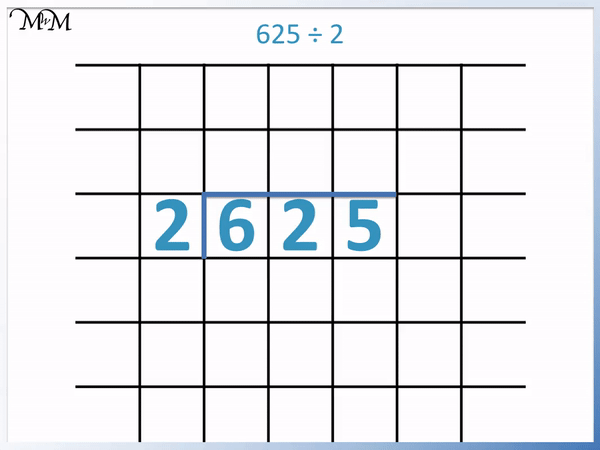• We have the short division of 625 ÷ 2.
• We divide each digit by 2 separately working from left to right.
• 6 ÷ 2 = 3.
• 2 ÷ 2 = 1.
• 2 does not divide exactly into 5.
• 2 × 2 = 4 which is 1 less than 5.
• 2 goes into 5 twice, with 1 left over.
• 5 ÷ 2 = 2 remainder 1.
• We can write 5 ÷ 2 = 2 r1.Supporting Lessons# The Short Division Method with Remainders

## What are remainders?

In maths, a remainder is the number that is left over after a division. A remainder is the difference between the number being divided and the largest multiple less than this number.

For example, 7 ÷ 3 = 2 remainder 1, because 3 × 2 = 6 and the difference between the number 7 and 6 is 1.

We write ‘remainder’ as ‘r’ for short. We write this r in before the remainder value.

So we can write 7 ÷ 3 = 2 remainder 1 as:

7 ÷ 3 = 2 r 1We can see that if we share 7 sweets equally amongst 3 people, they each get 2 sweets but there is 1 left over. We call this extra 1 a remainder. We have a remainder of 1.

## Short Division with Remainders

We will look at how to perform larger divisions with remainders by using the short division method.

In this example we have 625 ÷ 2.

We write the number being divided with a line to the left and above of it. The shape of this line often means that this method is called the ‘bus stop method’ for division.

We will divide each digit in the number 625 by 2 separately.We work from left to right, starting with the 6.6 ÷ 2 = 3

We write the 3 above the 6 in the short division.2 ÷ 2 = 1

We write the 1 above the 2.

We now look at the final digit.

2 does not divide exactly into 5.

2 × 2 = 4 and we need 1 more to make 5.

5 ÷ 2 = 2 r12 divides into 5 twice, with 1 left over. We write the 2 above the 5.

There are no more digits to divide by, so we write the leftover 1 as a remainder at the end of our answer.

We write this remainder as ‘r1’.Therefore,

625 ÷ 2 = 312 r 1.

Here is another example of short division involving remainders: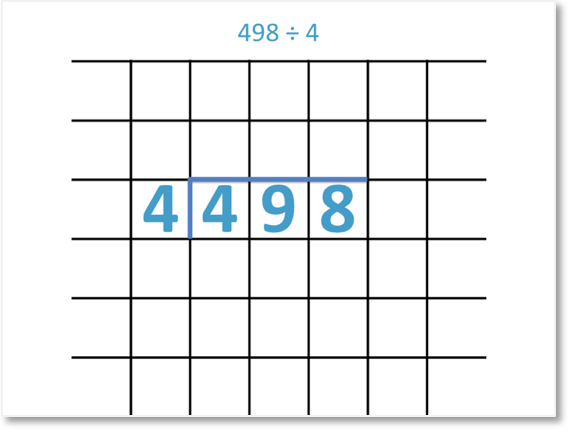We set out the short division calculation as shown above. We want to divide each digit in 498 by 4.

We work from left to right, starting with the 4.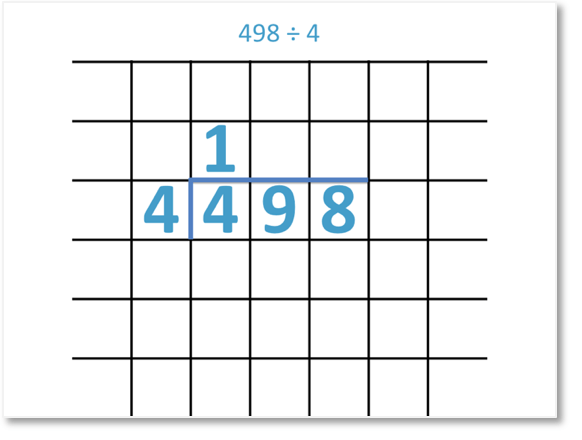4 ÷ 4 = 1

We write the 1 above the 4 in our division.9 ÷ 4 = 2

4 divides into 9 twice with 1 left over.

We write the 2 above the 9 and write the leftover 1 in front of the next digit along.

We now have 18 ÷ 4.18 ÷ 4 = 4

4 divides into 18 4 times, with 2 left over. We write the 4 above the 18.

There are no more digits to divide by, so we can write the leftover 2 as a remainder at the end of our answer.

We write the remainder as ‘r2’, which stands for ‘remainder 2’.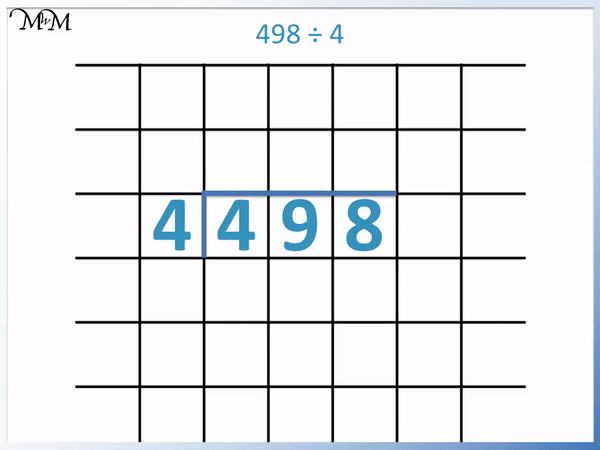Therefore,

498 ÷ 4 = 124 r2.

## Checking the Answer to a Short Division Problem

To check the answer to a division problem, multiply the answer by the number you have divided by. If the answer is correct, the result will be the original number that was divided.

If there is a remainder, multiply the whole number part of the answer by the number you divided by and then add the remainder on separately at the end.

In the example above, we divided 498 by 4 and got an answer of 124 r2.

To check the result we multiply the whole part of the answer by 4 and then add on the remainder.

4 × 124 = 496 and adding the remainder of 2, we get 498.

This is the original number that we divided in the question and so, this division is correct.

In this next example of short division with remainders we have 814 ÷ 3.

We divide each digit in 814 separately by 3.

8 ÷ 3 = 2 with 2 left over. This is because 3 goes into 8 twice to make 6. We need to add 2 more to 6 to get to 8.

We carry this ‘2’ over to the ‘1’ in the next column to make ’21’.

21 ÷ 3 = 7. This divides exactly and so, there is no remainder to carry.

Finally 4 ÷ 3 = 1 r1. This is because 3 goes into 4 once with 1 left over.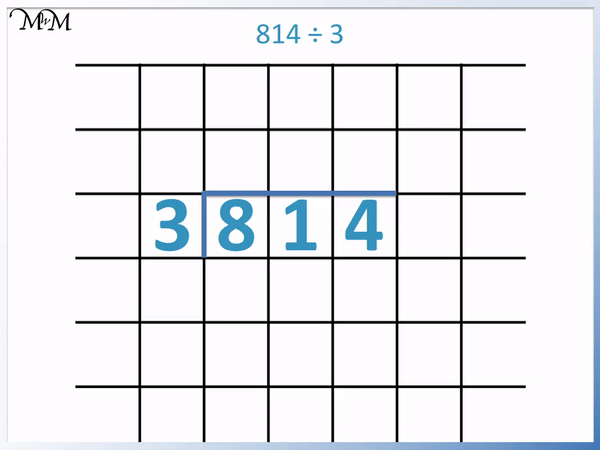We have divided all of the digits and so, this remainder of 1 is written at the end of the answer.

814 ÷ 3 = 271 r1.

To check the answer to this division problem, we multiply 271 by 3 and add the remainder on at the end.

271 × 3 = 813 and adding the remainder of 1 we get 814.

This is the original number that we started with and so, the division is correct.

In this next example of short division with remainders we have 819 ÷ 5.

8 ÷ 5 = 1 r3 because 5 goes into 8 once with 3 more needed to get to 8.

We carry the remainder of ‘3’ over to the next digit along ‘1’ to make ’31’.

31 ÷ 5 = 6 remainder 1 because 5 × 6 = 30 and 1 more is required to make 31.

We carry this remainder of ‘1’ over to the ‘9’ to make ’19’.

19 ÷ 5 = 3 remainder 4 because 5 × 3 = 15 and 4 more is needed to make 19.We have divided all of the digits in the number and so, we write the remainder at the end of our answer.

819 ÷ 5 = 163 remainder 4, which we can write as 163 r4.

To check the answer to this short division problem, we can multiply 163 by 5 and then add on the remainder of 4.

163 × 5 = 815 and adding the remainder of 4 makes 819.

This is the original number that we were dividing and so, the answer to the division is correct.Now try our lesson on Short Division: Remainders as Fractions where we learn how to write the remainders as fractions.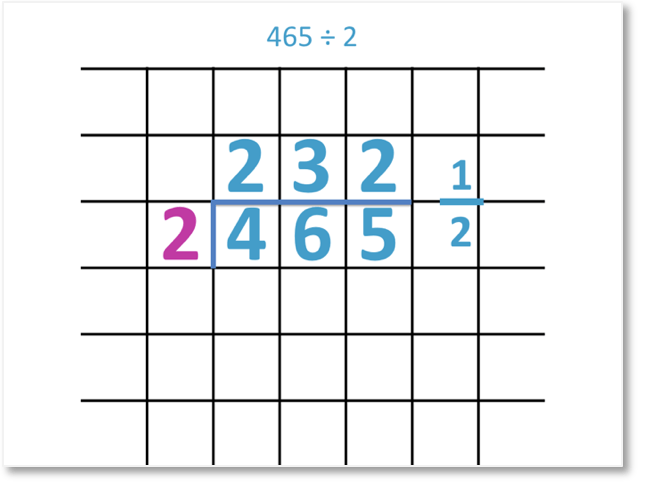error: Content is protected !!